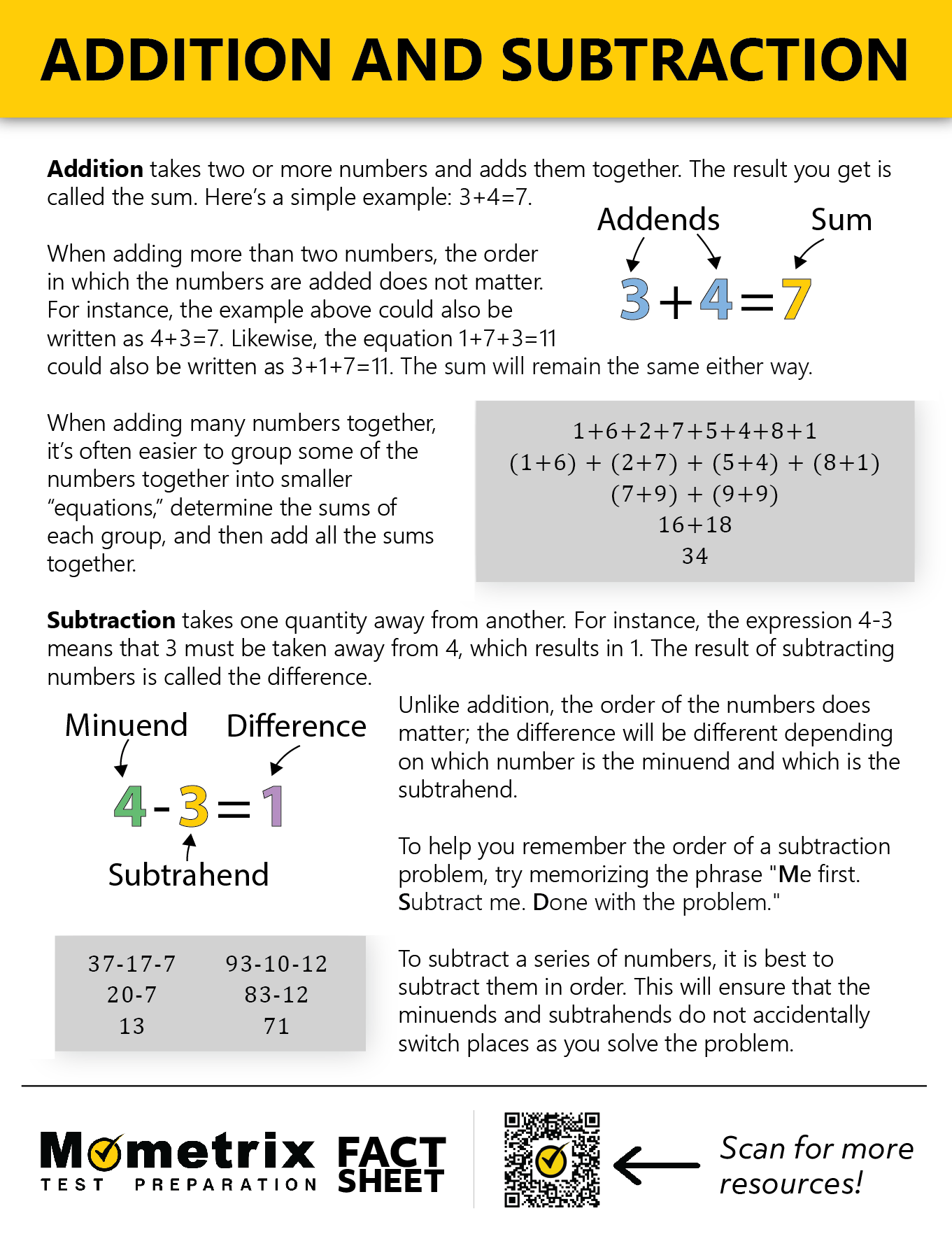# Solving Equations by Using Addition and Subtraction

### How do you solve an equation using the addition method?

#### A

To solve an equation, the variable needs to be isolated. In many equations, the variable can be isolated using addition. Remember, the number you are adding needs to be added to both sides of the equation in order to keep it balanced.

For example, the equation $$x-9=3$$ can be solved simply by adding $$9$$ to both sides of the equation. When $$9$$ is added to the left side, $$-9$$ and $$+9$$ cancel out, and $$x$$ is left on its own. When $$9$$ is added to the right side, $$3$$ becomes $$12$$. Now the equation shows $$x=12$$.

### What are the 4 steps to solving an equation?

#### A

1. Identify the variable. (usually $$x$$)
2. Combine “like-terms” if possible. For example, $$3+x=-9+4$$ would simplify to $$3+x=-5$$.
3. Attempt to isolate the variable by doing the same operation to both sides of the equation.
4. If a number is being added to the variable $$(x+5=9)$$ then use subtraction to solve. If a number is being subtracted from the variable $$(x-5=9)$$ then use addition to solve.

### How do you solve one-step equations with addition?

#### A

The goal of solving one-step equations with addition is to isolate the variable. In order to keep an equation balanced, the same number needs to be added to both sides of the equation. When solving an equation like $$m-4=17$$, the variable $$m$$ will be isolated if $$4$$ is added to both sides of the equation. $$m-4=17$$ becomes $$m=21$$.

### How do you solve linear equations?

#### A

The goal of solving a linear equation is to get the variable by itself. In order to do this, we need to “undo” whatever has been done to the variable. For example, in the equation $$x+5=8$$, five has been added to $$x$$. To “undo” this addition of five, we need to subtract five. If we subtract five from one side of the equation, we also must subtract five from the other side in order to keep the equation balanced. This “undo” process is referred to as inverse operations. The inverse of $$+5$$ would be $$-5$$. The inverse of $$-7$$ would be $$+7$$. In the equation $$x+5=8$$, $$x=3$$ when five is subtracted from both sides. This means that if $$3$$ is plugged into the equation, both sides will be equal: $$(\bf3)+5=8$$.

### How do you solve linear equations with subtraction?

#### A

Linear equations can often be solved with subtraction. For example, the equation $$9=4+x$$ can be solved by simply subtracting $$4$$ from both sides. The goal of solving a linear equation is to isolate the variable.

Consider the equation $$x+15=30$$. In order to isolate the variable, the $$+15$$ needs to be eliminated. This is achieved by subtracting $$15$$ from both sides of the equation. $$x+15=30$$ becomes $$x=15$$. This means that if $$15$$ is plugged in for $$x$$, the equation will be balanced: $$(15)+15=30$$.

#### A

Many equations are solvable using addition or subtraction. If a number is being added to a variable, subtraction will be used to “undo” this operation. If a number is being subtracted from a variable, addition will be used to “undo” this operation.

For example, $$y+2=7$$ can be solved by subtracting $$2$$ from both sides of the equation. $$y+2=7$$ becomes $$y=5$$. Other equations such as $$x-5=8$$ can be solved using addition. When $$5$$ is added to both sides, the equation becomes $$x=13$$.

### How do you solve two-step equations with addition?

#### A

To solve a two-step equation with addition, start by combining any “like-terms”. For example, the equation $$4+x-5=-9+1$$ would be simplified to $$x-1=-8$$ by combining like-terms. Now the goal is to isolate the variable in order to solve for $$x$$. In order to get $$x$$ alone, $$1$$ needs to be added to both sides of the equation. The equation now becomes $$x=-7$$. This two-step process works regardless of $$x$$ being on the left or right side of the equation. The two steps to remember are combine like-terms, and isolate the variable.

## Fact Sheet## Practice Questions

Question #1:

$$2x+13=41$$

$$x=27$$
$$x=7$$
$$x=14$$
$$x=28$$

The correct answer is $$x=14$$.
$$2x+13=41$$
The first thing to do with this equation is subtract 13 from both sides.
$$2x=28$$
Then, divide both sides by 2.
$$x=14$$

Question #2:

$$8x-29=14$$

$$x=-1$$
$$x=5$$
$$x=-1\frac{7}{8}$$
$$x=5\frac{3}{8}$$

The correct answer is $$x=5\frac{3}{8}$$.
$$8x-29=14$$
The first thing to do with this equation is add 29 to both sides.
$$8x=43$$
Then, divide both sides by 8.
$$x=\frac{43}{8}$$
Finally, turn $$\frac{43}{8}$$ into a mixed number.
$$x=5\frac{3}{8}$$

Question #3:

$$41-2x=19$$

$$x=30$$
$$x=11$$
$$x=-30$$
$$x=-11$$

The correct answer is $$x=11$$.
$$41-2x=19$$
The first thing to do with this equation is subtract 41 from both sides.
$$-2x=-22$$
Then, divide both sides by -2.
$$x=11$$

Question #4:

$$21+13-x=3x-6$$

$$x=10$$
$$x=11$$
$$x=14$$
$$x=9$$

The correct answer is $$x=10$$.
$$21+13-x=3x-6$$
The first thing to do with this equation is combine like terms.
$$34-x=3x-6$$
Next, add 6 to both sides.
$$40=4x$$
Finally, divide both sides by 4.
$$10=x$$

Question #5:

$$x-17+11=21-5$$

$$x=22$$
$$x=10$$
$$x=14$$
$$x=27$$

The correct answer is $$x=22$$.
$$x-17+11=21-5$$
The first thing to do with this equation is combine like terms.
$$x-6=16$$
Then, add 6 to both sides.
$$x=22$$

447444

by Mometrix Test Preparation | This Page Last Updated: August 2, 2023

Get Actionable Study Tips
Join our newsletter to get the study tips, test-taking strategies, and key insights that high-performing students use.
Get Actionable Study Tips
Join our newsletter to get the study tips, test-taking strategies, and key insights that high-performing students use!